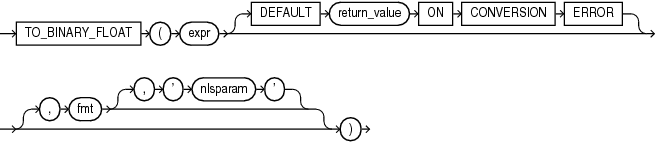## TO_BINARY_FLOAT

SyntaxDescription of the illustration to_binary_float.eps

Purpose

`TO_BINARY_FLOAT` converts `expr` to a single-precision floating-point number.

• `expr` can be any expression that evaluates to a character string of type `CHAR`, `VARCHAR2`, `NCHAR`, or `NVARCHAR2`, a numeric value of type `NUMBER`, `BINARY_FLOAT`, or `BINARY_DOUBLE`, or null. If `expr` is `BINARY_FLOAT`, then the function returns `expr`. If `expr` evaluates to null, then the function returns null. Otherwise, the function converts `expr` to a `BINARY_FLOAT` value.

• The optional `DEFAULT` `return_value` `ON` `CONVERSION` `ERROR` clause allows you to specify the value returned by this function if an error occurs while converting `expr` to `BINARY_FLOAT`. This clause has no effect if an error occurs while evaluating `expr`. The `return_value` can be an expression or a bind variable, and must evaluate to a character string of type `CHAR`, `VARCHAR2`, `NCHAR`, or `NVARCHAR2`, a numeric value of type `NUMBER`, `BINARY_FLOAT`, or `BINARY_DOUBLE`, or null. The function converts `return_value` to `BINARY_FLOAT` in the same way it converts `expr` to `BINARY_FLOAT`. If `return_value` cannot be converted to `BINARY_FLOAT`, then the function returns an error.

• The optional '`fmt`' and '`nlsparam`' arguments serve the same purpose as for the `TO_NUMBER` function. If you specify these arguments, then `expr` and `return_value`, if specified, must each be a character string or null. If either is a character string, then the function uses the `fmt` and `nlsparam` arguments to convert the character string to a `BINARY_FLOAT` value.

If `expr` or `return_value` evaluate to the following character strings, then the function converts them as follows:

• The case-insensitive string '`INF`' is converted to positive infinity.

• The case-insensitive string '-`INF`' is converted to negative identity.

• The case-insensitive string '`NaN`' is converted to `NaN` (not a number).

You cannot use a floating-point number format element (`F`, `f`, `D`, or `d`) in a character string `expr`.

Conversions from character strings or `NUMBER` to `BINARY_FLOAT` can be inexact, because the `NUMBER` and character types use decimal precision to represent the numeric value and `BINARY_FLOAT` uses binary precision.

Conversions from `BINARY_DOUBLE` to `BINARY_FLOAT` are inexact if the `BINARY_DOUBLE` value uses more bits of precision than supported by the `BINARY_FLOAT`.

Examples

Using table `float_point_demo` created for TO_BINARY_DOUBLE, the following example converts a value of data type `NUMBER` to a value of data type `BINARY_FLOAT`:

```SELECT dec_num, TO_BINARY_FLOAT(dec_num)
FROM float_point_demo;

DEC_NUM TO_BINARY_FLOAT(DEC_NUM)
---------- ------------------------
1234.56               1.235E+003```

The following example returns the default value of `0` because the specified expression cannot be converted to a `BINARY_FLOAT` value:

```SELECT TO_BINARY_FLOAT('2oo' DEFAULT 0 ON CONVERSION ERROR) "Value"
FROM DUAL;

Value
----------
0
```